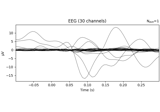# mne.decoding.UnsupervisedSpatialFilter¶

class `mne.decoding.``UnsupervisedSpatialFilter`(estimator, average=False)[source]

Use unsupervised spatial filtering across time and samples.

Parameters
estimatorinstance of `sklearn.base.BaseEstimator`

Estimator using some decomposition algorithm.

averagebool, default `False`

If True, the estimator is fitted on the average across samples (e.g. epochs).

Methods

 `__hash__`(/) Return hash(self). `fit`(X[, y]) Fit the spatial filters. `fit_transform`(X[, y]) Transform the data to its filtered components after fitting. `get_params`([deep]) Get parameters for this estimator. Inverse transform the data to its original space. `set_params`(**params) Set the parameters of this estimator. Transform the data to its spatial filters.
`fit`(X, y=None)[source]

Fit the spatial filters.

Parameters
X`array`, shape (n_epochs, n_channels, n_times)

The data to be filtered.

y`None` | `array`, shape (n_samples,)

Used for scikit-learn compatibility.

Returns
selfinstance of `UnsupervisedSpatialFilter`

Return the modified instance.

`fit_transform`(X, y=None)[source]

Transform the data to its filtered components after fitting.

Parameters
X`array`, shape (n_epochs, n_channels, n_times)

The data to be filtered.

y`None` | `array`, shape (n_samples,)

Used for scikit-learn compatibility.

Returns
X`array`, shape (n_epochs, n_channels, n_times)

The transformed data.

Examples using `fit_transform`:`get_params`(deep=True)[source]

Get parameters for this estimator.

Parameters
deepbool, optional

If True, will return the parameters for this estimator and contained subobjects that are estimators.

Returns
params`dict`

Parameter names mapped to their values.

`inverse_transform`(X)[source]

Inverse transform the data to its original space.

Parameters
X`array`, shape (n_epochs, n_components, n_times)

The data to be inverted.

Returns
X`array`, shape (n_epochs, n_channels, n_times)

The transformed data.

`set_params`(**params)[source]

Set the parameters of this estimator.

The method works on simple estimators as well as on nested objects (such as pipelines). The latter have parameters of the form `<component>__<parameter>` so that it’s possible to update each component of a nested object.

Parameters
**params`dict`

Parameters.

Returns
instinstance

The object.

`transform`(X)[source]

Transform the data to its spatial filters.

Parameters
X`array`, shape (n_epochs, n_channels, n_times)

The data to be filtered.

Returns
X`array`, shape (n_epochs, n_channels, n_times)

The transformed data.

## Examples using `mne.decoding.UnsupervisedSpatialFilter`¶